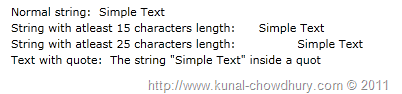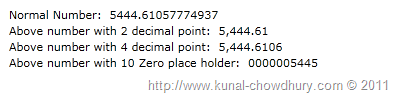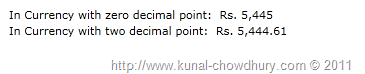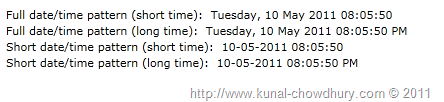# Formatting text in Silverlight XAML using StringFormat

In my latest two tips we have seen that we can customize the string using Run, Span tags as well as StringFormat in the XAML page. What abou... - Article authored by Kunal Chowdhury on .

In my latest two tips we have seen that we can customize the string using Run, Span tags as well as StringFormat in the XAML page. What about if I want to format it for proper DateTime, Currency or Numeric style? As I told you in my last post that, we can achieve the same using the StringFormat parameter in XAML too.

Here in this post, I will demonstrate you the process in depth. Read to know more about it and make your life more comfortable designing your Silverlight application.

If you didn't read my previous two tips, you can find them here:

## Working with Strings

Let us first see what we can do using StringFormat for our strings? We can use various string formats mentioned here in MSDN document. Take reference from it if you want. So, start with a simple example.

At the first step, we will create a DependencyProperty of type string in our code behind called "Text". Then we will add the following code in our XAML page:

` `
`<TextBlock>`
`    <Run Text="Normal string: "/>`
`    <Run Text="{Binding Text, ElementName=userControl}"/>`
`</TextBlock>`
`<TextBlock>`
`    <Run Text="String with atleast 15 characters length: "/>`
`    <Run Text="{Binding Text, StringFormat=\{0\,15\}, ElementName=userControl}"/>`
`</TextBlock>`
`<TextBlock>`
`    <Run Text="String with atleast 25 characters length: "/>`
`    <Run Text="{Binding Text, StringFormat=\{0\,25\}, ElementName=userControl}"/>`
`</TextBlock>`
`<TextBlock>`
`    <Run Text="Text with quote: "/>`
`    <Run Text="{Binding Text, StringFormat='The string &quot;\{0\}&quot; inside a quot',`
`                              ElementName=userControl}"/>`
`</TextBlock>`

The first TextBlock will show a normal string in the UI. The 2nd one will have total 15 characters length and if our text is less than 15, it will show a blank space of the remaining length at the front. The 3rd example will show the same case but for 25 characters. In this case, it will have more space at the front. The 4th example will show a concatenated string in the UI with a quot. Remember that, you have to specify a valid ASCII value there.

The above code will render the following UI in the screen:## Working with Numbers

As shown above, you can format a number too. Read the MSDN document here to know more about the format of the same.

First of all, create a DependencyProperty for our example which will return a numeric value. Now, use the below example to learn about it's use in XAML:

` `
`<TextBlock>`
`    <Run Text="Normal Number: "/>`
`    <Run Text="{Binding Number, ElementName=userControl}"/>`
`</TextBlock>`
`<TextBlock>`
`    <Run Text="Above number with 2 decimal point: "/>`
`    <Run Text="{Binding Number, StringFormat=\{0:n2\}, ElementName=userControl}"/>`
`</TextBlock>`
`<TextBlock>`
`    <Run Text="Above number with 4 decimal point: "/>`
`    <Run Text="{Binding Number, StringFormat=\{0:n4\}, ElementName=userControl}"/>`
`</TextBlock>`
`<TextBlock>`
`    <Run Text="Above number with 10 Zero place holder: "/>`
`    <Run Text="{Binding Number, StringFormat=\{0:0000000000\}, ElementName=userControl}"/>`
`</TextBlock>`

We used {0:nx} to format a numeric value where 'n' formats it as numeric and 'x' represents no. of decimal point.

In this example, we will 1st see the number in normal format. The 2nd example will show the same number in two decimal point. The 3rd example showcases it with 4 decimal point. The 4th one puts zero as place holder of 10 digits. If the number is less than the specified length, it will show zero's preceding the original no.

Let's have a demo of the above code here:You can see here that, as the original no. is of 4 digits, it adds 6 zeros in front of the no. to make it a 10 digit number.

## Working with Currencies

Let's have a demo on currency too. This will localize the currency based on the rule already set in the client system. We will use the same property used in the previous point.

We will use the following XAML code to format our currency value, to demonstrate the example:

` `
`<TextBlock>`
`    <Run Text="In Currency with zero decimal point: "/>`
`    <Run Text="{Binding Number, StringFormat=\{0:c0\}, ElementName=userControl}"/>`
`</TextBlock>`
`<TextBlock>`
`    <Run Text="In Currency with two decimal point: "/>`
`    <Run Text="{Binding Number, StringFormat=\{0:c2\}, ElementName=userControl}"/>`
`</TextBlock>`

We will format the Number as Currency by specifying the StringFormat as {0:cx} where 'c' defines the currency format and 'x' represents the decimal point. In the 1st TextBlock, we will have a currency with zero decimal point and in the 2nd one will have a two decimal point.

Let's see the demo of the above example here:As the above example was run in my machine (in India), hence you can notice that it shows the value in Indian currency format (Rs.). If you run it in different location having a different localization, it will show the currency in that format only.

## Working with DateTime

Working with DateTime is also similar to that. If you are familiar with the formats mentioned in MSDN documentation, you can easily format your DateTime accordingly. Find the valid formats for DateTime here. I am not going to discuss more on the format but will give you some example which you can use to apply different styles.

Let us use the following XAML code:

` `
`<TextBlock>`
`    <Run Text="Full date/time pattern (short time): "/>`
`    <Run Text="{Binding DateTime, StringFormat=f, ElementName=userControl}"/>`
`</TextBlock>`
`<TextBlock>`
`    <Run Text="Full date/time pattern (long time): "/>`
`    <Run Text="{Binding DateTime, StringFormat=F, ElementName=userControl}"/>`
`</TextBlock>`
`<TextBlock>`
`    <Run Text="Short date/time pattern (short time): "/>`
`    <Run Text="{Binding DateTime, StringFormat=g, ElementName=userControl}"/>`
`</TextBlock>`
`<TextBlock>`
`    <Run Text="Short date/time pattern (long time): "/>`
`    <Run Text="{Binding DateTime, StringFormat=G, ElementName=userControl}"/>`
`</TextBlock>`

The 1st example will show the DateTime in full date and short time format, the 2nd example show both of them in long format. 3rd example will show both the date and time in short format and the 4th one will format the date in short pattern and the time in long pattern.

See the demonstration of the above code here:All these values depends on your machine configuration. Hence, you may notice a different thing based on your localization settings in your computer.

## End Note

Hope this post is helpful to you and you understood how to format a string in XAML using the StringFormat parameter while binding the data. Download the full solution from here if you want to run it and use the code.

Don't forget to leave your feedback at the end of the post. Suggestions are always welcome. I also tweets. Follow me on twitter @kunal2383. Thanks for reading this post. Happy Coding.

## Have a question? Or, a comment? Let's Discuss it below...

Thank you for visiting our website!